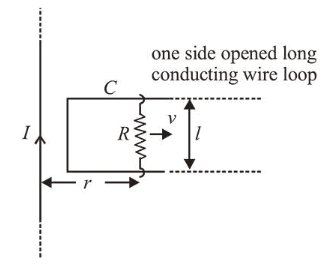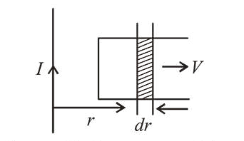# An infinitely long straight wire carrying current I,

Question:

An infinitely long straight wire carrying current $I$, one side opened rectangular loop and a conductor $C$ with a sliding connector are located in the same plane, as shown in the figure. The connector has length $l$ and resistance $R$. It slides to the right with a velocity $v$. The resistance of the conductor and the self inductance of the loop are negligible. The induced current in the loop, as a function of separation $r$, between the connector and the straight wire is :1. (1) $\frac{\mu_{0}}{4 \pi} \frac{I v l}{R r}$

2. (2) $\frac{\mu_{0}}{\pi} \frac{I v l}{R r}$

3. (3) $\frac{2 \mu_{0}}{\pi} \frac{I v l}{R r}$

4. (4) $\frac{\mu_{0}}{2 \pi} \frac{I v l}{R r}$

Correct Option: 4,

Solution:

(4) Magnetic field at a distance $r$ from the wire

$B=\frac{\mu_{0} I}{2 \pi r}$

Magnetic flux for small displacement $d r$;

\phi=B \cdot A=B l d r \quad\left[\because A=l d r\right.$and$\left.B \cdot A=B A \cos 0^{\circ}\right]\Rightarrow \phi=\frac{\mu_{0} I}{2 \pi r} l d r$Emf,$e=\frac{d \phi}{d t}=\frac{\mu_{0} I l}{2 \pi r} \cdot \frac{d r}{d t} \Rightarrow e=\frac{\mu_{0}}{2 \pi} \cdot \frac{I v l}{r}$Induce current in the loop,$i=\frac{e}{R}=\frac{\mu_{0}}{2 \pi} \cdot \frac{I v l}{R r}\$